Pathways To Learning... Since 2005 Hong Kong Registered School 566985

# A - An to azimuth - Mathematics Dictionary

A   B   C   D   E   F   G   H   I   J   K   L   M   N   O   P   Q   R   S   T   U   V   W   X   Y   Z

An : The alternating group of degree n and order n!/2. That is, a group has n!/2 members which acts on n elements of a set. It is a group that consists of members which are even permutations (compositions of even number of permutations) only.

Abelian group: A commutative group. class="d-title" Named after Norwegian mathematician Niels Henrik Abel. It is a group for which the order of applying its elements does not matter (commutativity). It is often written in lower case nowadays.

Abel's test: A sufficient but not necessary criterion for the convergence of a series. A convergent series an and a bounded monotonic sequence bn imply that the infinite series a1b1 + a2b2+ ... +anbn+ . .. is convergent.

abridged multiplication: A method of multiplication where digits may be dropped in each step of the calculation, in order to simplify the calculations, while maintaining the desired level of accuracy, usually expressed in terms of decimal places.

abscissa: (plural abscissae) A somewhat obsolete term for the x-coordinate. In a rectilinear coordinate system (i.e. Cartesian ), it is measured from the y-asis parallel to the x-axis. Compare ordinate.

absolute error: The difference between two values, always taken to be positive. For example, the difference between an observed/inferred value and the theoretical/actual value.

absolutely convergent series: A convergent series which remains convergent even if the modulus function is applied to each individual term before summation.

absolute maximum (minimum): Values in a set of numbers which are, depending on context, greater than(less than), or not less than(not greater than), any other values in the set of numbers, e.g. values of a function, terms of a sequence.

absolute number: 1. A real value with its sign ignored - the result of applying the modulus function to a value. Also known as absolute value.

2. A known constant, usually represented by numerals.

absolute term: The constant term in an expression such as a polynomial.

absolute value: 1. A real value with its sign ignored - the result of applying the modulus function to a value.

2. The result of applying the modulus function to a complex value.

3. The magnitude of a vector.

absolute value rules: General results deduced for the modulus function, as applied to complex numbers:

1. |z| = |-z|

2. |z| ≥ 0 for all possible values of z

3. |xy| = |x| |y|

4. |x / y| = |x| / |y|

5. | (|x| - |y|) | ≤ |x + y| ≤ |x| + |y| [This is known as the Triangle Inequalities]

acceleration: The term describes two related concepts:

1. The vector quantity that is the rate of change of velocity (also a vector quantity).

2. The scalar quantity that is the rate of change of speed (also a scalar quantity).

acceleration of free fall: The acceleration due to gravity, which varies depending on the distance to the centre of the Earth. In most cases, taken to be constant, with the value at sea level.

acceptance region: The set of values for a random variable in hypothesis testing such that the null hypothesis is not rejected.

acceptance sampling: A method of make a decision of acceptance or rejection of a batch of items by randomly sampling a portion of it. A decision is taken by comparing the proportion of defective samples against an acceptable proportion beforehand. It is a necessary method when the items perish under testing and yet ensuring that the items are of sufficient high quality is important.

accumulation point: Also known as a limitpoint.

accuracy: A measure of how little an approximation deviates from the true value.

action: 1. A quantity of a dynamical system, the study of which sheds light onto the tendency of the evolution of such a system.

2. A force. In the context of Newton's Third Law and another force (the reaction) that it induces.

acute angle: An angle less than that of a right angle. (The trivial case of an angle of 0° is excluded.)

acute triangle: A triangle for which all three angles are acute (i.e. between 0° and 90°).

addition: A binary mathematical operation that represents the abstract concept of the formation of a larger collection (sum) from two smaller collections (the addends/operands). Operated on the natural numbers, this represents the cardinality of the the union of two sets of distinct members. Addition is then extended in its definition to cover all integers, rational numbers, algebraic numbers, real numbers and complex numbers as well as polynomials.

addition formulae: Also known as Compound Angle Formulae. Since trigonometric functions are not linear, they express the trigonometric ratio of sums of angles as functions of individual trigonometric ratios of the component angles:

sin (A + B) = sin (A) cos (B) + cos (A) sin (B)

cos (A + B) = cos (A) cos (B) – sin (A) sin (B)

tan (A + B) = (tan (A) + tan (B))/(1 – tan (A) tan (B))

The subtraction formulae, accordingly, are:

sin (A - B) = sin (A) cos (B) - cos (A) sin (B)

cos (A - B) = cos (A) cos (B) + sin (A) sin (B)

tan (A – B) = (tan (A) – tan (B))/(1 + tan (A) tan (B))

additive function: A function whose value on the sum of two arguments is the same as the sum on the values of the arguments, regardless of the choice of the arguments.

For all values of x and y, f (x+y) = f (x) + f (y)

ad infinitum: Literally meaning "to infinity" in Latin. It is a phrase used in mathematics (and other fields) as a more elegant way of what is more commonly expressed as "... and so on and so forth".

adjacent: A concept of objects being located "next to each other" or "without anything else in between"

1. For objects of different types (an edge and an angle, an edge and a vertex etc.), they are considered adjacent if there is no "space" between the two. (Usually used informally.)

1. For angles (in geometry), they are adjacent if they share an edge (side) in their constituent edges which form the angles.

2. For vertices (in geometry and graph theory), they are adjacent if connected by an edge.

3. For edges (in geometry and graph theory). they are adjacent if separated by a vertex.

4. For faces (in geometry), they are adjacent if separated by an edge.

adjoint (of a matrix): The transpose of the cofactor matrix for a square matrix. It is also the result of multiplying the inverse matrix by the determinant of the original matrix.

affine transformation: A composition of linear transformations and translations. It can be thought of as a sort of linear transformation where all properties related to the origin being regarded as a special point no longer exists. Alternatively it can be thoght of as a transformation that is a combination of translations, projections, rotations, reflections, stretches and shrinks (thereby also including enlargements).

aggregation: The process of representing the sum of mathematical terms as a single (mathematical) term.

algebra: A branch of mathematics which studies the properties and resulting structures from applying operations on mathematical objects. While the motivation originates from the study of unknown numbers, algebra in modern mathematics has evloved to deal with a variety of abstract mathematical constructions.

algebraic curve: A collection of points, of one-dimension, which represents the solutions of a collection of polynomials.

algebraic expression: Is a mathematical phrase that is written using one or more variables and constants, but which does not contain a relation symbol (). Example: 5y + 8

algebraic number: An algebraic number is a number which is the root of a polynomial of integer coefficients. Note that all rational numbers are algebraic numbers. (solution of linear equations.) Although irrational numbers can be algebraic as well. (sqrt(2) is a root to the equation x2-2=0)

algorithm: A set of constructive instructions designed to solve a specific problem. In order to be considered an algorithm, the procedures should require no additional thoughts (heuristics). It can be thought of as instructions fit for implementation on machines (computers). The term is derived from an Arab mathematician who was known as al-Khwarizmi al-Haytham, Abu 'Ali ai-Hasan ibn (c.965—1038).

alternate angles: Angles which are essentially vertically opposite angles, while not sharing the same vertex, if we identify as the same the two intersections between a pair of parallel lines and another line. The more common form cited are more specifically known as interior alternate angles, alternate angles which are both inside of the pair of parallel lines, coloquially known as "Z-angles" (not an accepted term in most examinations). The other form are called exterior alternate angles accordingly, being both outside of the pair of parallel lines.2=4=6=8 and 1=3=5=7

alternate segment theorem: A circle theorem identifying the angle between a chord of 2 points and the tangent of one of the points, and the angle formed by the two points on the circumference in the "alternate segment", as the same. If the former angle is acute, then the latter angle is on the major arc; while the former angle being obtuse implies that the latter angle must be on the minor arc. See circle for more detail.

 alternating series: A series with terms (an) which strictly alternates between positive and negative values. The significance of this requirement lies in the ability to bound the sum of such an infinite series by successive partial sums, if the series is also (strictly) decreasing in magnitude, with each successive partial sum improves on the upper and lower bound alternatelyt. While a limit of zero for the sequence (dimishing terms) are a necessary but not sufficient condition for convergence for a series in general, it is both necessary and sufficient for convergence in the case of an alternating series.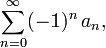alternative hypothesis: The model considered to be the case if the null hypothesis in considered to be rejected (not hold). There is some debate as to whether an alternative hypothesis increases the subjectiveness of hypothesis testing, since the alternative hypothesis does not necessarily have to be the negation of the null hypothesis. The issue is whether it is appropriate to make presumptions of what the model in consideration cannot be (models not covered by the null or alternative hypothesis but not considered) in hypothesis testing. Setting the alternative hypothesis to be the negation of the null hypothesis amounts to not using alternative hypothesis as some statistician would advocate.

altitude: 1. The line segment from the vertex of a shape, extending to the base of the same shape such that the line segment is perpendicular to the base. Also known as a perpendicular drop.

2. The length of such a line segment.

ambiguous case: Commonly refering to the situation in trigonometry where insufficient information are given in specifying a triangle (up to congruence), while appearing otherwise. While congruence conditions for triangles require two sides and an angle between the two given sides (SAS) amongst other possibilities, the ambiguity arises when two sides and an angle other than the one between the two sides is given.

It should be noted that "two sides and an angle not in between" (SSA, or ASS as it can be a helpful mnemomic for some) does not guarantee ambiguity. The so-called RHS or Right Angle-Hypotenuse-Side (UK and others), also known as LH or Leg-Hypotenuse (US and others) condition is one special case.

A unique triangle is determined if the angle given is larger than (or equal to) the angle subsequently found via sine rule (in the situation SSA - to find an angle), meaning that the ambiguous case arises when the angle given is less than the angle found. When using the cosine rule (in the situation SSA - to find a length), the ambiguous case presents itself in the form of two positive solutions from the quadratic equation that ensues the initial implementation of cosine rule.

amplitude: A measure of maximum displacement for an oscillatory system. For a sinusoidal curve, it is measured from the principal axis (midpoint between the maximum value and the minimum value).

analysis: A branch of mathematics that studies functions, sequences and related concepts. In a vague sense, the word "analysis" in the class="d-title" name suggests that the branch predominantly seeks to understand these "from within" rather than looking at their structure as a whole.

analytic methods: Methods for solving problems which involves breaking down and studying the consequences of the "internal" properties and/or components of objects involved in the problem, as opposed to solution primarily through its "external" relations to other objects.

and: A logical connective that captures the meaning of our understanding of the everyday word "and". It is used on 2 propositions (say, a and b) therefore it can be considered a binary operation. The proposition a^b (also written a&b - considered as a single proposition) is only true when both of its component propositions are true.angle: A measure of the "separation" of 2 lines (planes) by the minimal amount of rotation about the common intersection point (line) in order for one line (plane) to coincide with the other.

angle bisector theorem: A theorem which states that, given any triangle and any one of its angle, the angle bisector divides its opposite side into 2 segments whose length are of the same ratio as the lengths of the corresponding adjacent sides.

angular acceleration: The time derivative of angular velocity, that is, the rate (with respect of time) of increase of angular velocity.

angular frequency: Also known as angular speed. (So called because the measure is essentially the same as how often - frequency - a certain unit of angle is achieved.)

angular measure: The angle between the line from the observer to object 1 and the line from the observer to object 2. For example: Shortly after sunrise, you see that the sun is only a few degrees above the horizon, while at noon it is elevated to nearly 90 degrees (or directly overhead). You are the observer, the sun is Object 1, and the horizon is Object 2.

angular momentum: A measure of the state of a physical system that is related to its spin or abiliy to spin. Analogous to linear momentum, angular momentum of a system is conserved unless an external force is applied in a particular way.

angular velocity: Is the rate of change of the angular position of a rotating body; mainly expressed in radians per second or per minute.

antecedent: In the conventional way of expressing hypothetical propositions, "If A then B", the consequent is the first part of the sentence. It is the part of the sentence whose truth value is independent of the other part. (Within the context of the statement itself.)

antiderivative: Given a function, the function (or family of functions) whose derivative is the given function. It is also called the indefinite integral.

antidiagonal: For conventionally written matrices, the diagonal that runs from the top right to thr bottom left. i.e. The "other" diagonal, as opposed to the main diagonal. (The main diagonal is the diagonal that the elements "1" in an identity matrix runs along.) See matrix.

antidifferentiation: Also known as indefinite integration.

antiparallel vectors: A pair of vectors pointing in the exact opposite directions to each other.

antipodal points: The points that are diametrically opposite on a circle or a sphere. The definition also extends to an ellipse or an ellipsoid.

antisymmetric matrix: A matrix such that it's sum with its transpose matrix is the zero matrix.

antisymmetric relation: A binary relation ~ where if A is related to B (A~B) and B is related to A (B~A) then A must be identical to B.

apex: The vertex in a geometric figure furthest from the base. The plural of an apex is apices.

apothem: The segment of the perpendicular bisector of a side of a regular polygon that runs between the centre and the side.

arc length of a curve: The length of a segment of a curve. It can be thought of as the limit of the sum of the lengths of any type of curves (circular, straight or otherwise) that approximates the curve itself.

area: The extent or measurement of a surface or piece of land or the extent of a 2-dimensional surface enclosed within a boundary; "the area of a rectangle";

area formulae: Established formulae for calculation of simple shapes.

square = a2rectangle = a x bparallelogram = b x h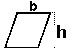trapezoid = h x (b1 + b2) / 2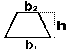circle =pi x r2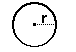ellipse = pi x r1 x r2area sampling: An example of clustered sampling where geographic information is used to form "natural" clusters.

arg: A unary operation on a complex number whose value (output) is the argument of the complex number (input). See argument (of a complex number).

 Argand diagram: A graphical representation of complex numbers that reflects certain internal structures, using the cartesian system where points on the diagram corresponds to complex numbers. i.e. the point (a,b) corresponds with the complex number a + bi.argument: 1. The "input" of a function.

2. The amount of rotation anticlockwise that the initial line (positive x axis) must make on an argand diagram to form the line that contains the complex number in question. If the angle is reflex then the argument is considered negative, and the absolute value of the argument is the amount of clockwise rotation instead.

arithmetic: A branch of mathematics which studies the computation of quantities using elementary operations.

arithmetic-geometric mean inequality: An inequality which states that the geometric mean of 2 positive numbers is always less than or equal to the arithmetic mean of the same 2 numbers.

(a+b)/2 ≥ √(ab)

Note that this result can be extended to any number of numbers and the term may refer to these results as well.

arithmetic mean: An average value of a set of numbers calculated by dividing the sum of the set of numbers by the number of numbers in the set.

arithmetic progression: A sequence where consecutive terms differ by the same amount, that is, the directed difference between each term and the next is always the same.

arm: A half-line extending from a vertex or the centre (of a circle, sphere, ellipse etc.) or origin.

array: A configuration or arrangement of objects which is considered systematic in some ways, including but not limited to a rectangular array, where matrices (and by extension, the matrix representation of vectors) are examples.

association: A binary relation held by two dependent variables in statistics. Two variables are not associated if and only if they are independent of each other.

associative: Describes the property of any binary operation (in this case, °) where the order, in which the multitude of identical operation to be performed, does not matter. (Not to be confused with the property of commutativity where the order of the arguments to an operation does not matter.) Alternatively, it is the property where the associative law applies,

a ° (b ° c) = (a ° b) ° c

In this case, the expression would usually be written as a ° b ° c (without any parentheses), since the order in which the operation is performed, does not matter.

Addition is one common operation in which associativity applies,

a + (b + c) = (a + b) + c

as is the case for multiplication,

a(bc) = (ab)c

asymmetric relation: A binary relation that is not symmetric.

asymmetry: The property of not having any symmetry. (Within context, so that we can still talk about the asymmetry of symmetric objects.)

asymptote: The relation between 2 curves whose distance becomes arbitrarily small outside any neighbourhood of any intersection of the curves. Although the word is usually used when one such curve is a line, in which case the line is considered the asymptote to the curve. (And seldom the other way around.)

asymptotic: 1. Of or related to an asymptote, having an asymptote or capable of having an asymptote.

2. The relation between two functions that tends to the same value (possibly infinite) and such that the difference between the values of the 2 functions, considered as a proportion of the values of either function, becomes arbitrarily small.

a
 asymptotic series: An infinite series whose partial sums become increasingly good approximations of the limit as the argument tends to infinity.augend: An argument in the binary operation of addition. Technically the second argument, it is commonly applied to both arguments due to addition being commutative. See addition.

 augmented matrix: A matrix formed by appending two matrices together. One application is to solve a system of linear equations by rewriting it as an augmented matrix before applying row operations.This augmented matrix essentially combines the two known matrices in the matrix equation that is equivalent to the system of linear equations, by simply writing the 2 matrices side by side and considered as one matrix.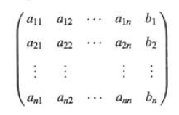auxiliary circle: 1. The smallest circle which circumscribed an ellipse. Thus, the circle has the same centre as the ellipse and the radius of the circle is the length of the semimajor axis.

2. The largest circle whose centre lies on the centre (the intersection of the lines of symmetry and/or the asymptotes) of the hyperbola.

auxiliary equation: A polynomial formed by tranforming a linear differential equation with a substitution. This, for example, transforms the problem of solving a second order linear differential equation into the problem of solving a quadratic equation

am2 + bn + c = 0

 The above equation is the auxiliary equation of the second order linear differential equation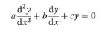average: A number of different but related concepts which corresponds to the mathematical/statistical idea of central tendency. Common taken to be the mean if unspecified.

axiom: A statement that is neither proven, nor is it intended to be proven. Usually they are considered to be self-evident, although axioms are also constructed to explore the possibilities of hypothetical situations where we accept certain assertions to be true, and what theorems could be derived from it.

axis: A line of reference regarding a mathematical object. The plural form of the word axis is "axes".

1.Coordinate system - a (straight) line from which (or a combination of such lines) the distance/orientation of an object is used to refer to the location of the object.

2. Transformation (rotation or reflection) - a (straight) line formed by the invariant points of the transformation.

azimuth: The angle between the projection of the radius vector onto the plane perpendicular to the polar axis, and the initial line.

A   B   C   D   E   F   G   H   I   J   K   L   M   N   O   P   Q   R   S   T   U   V   W   X   Y   Z

Genius is one percent inspiration and ninety-nine percent perspiration.